# 添加简单的光# 光的类型

L=Q-P

L=Q

## 物体反射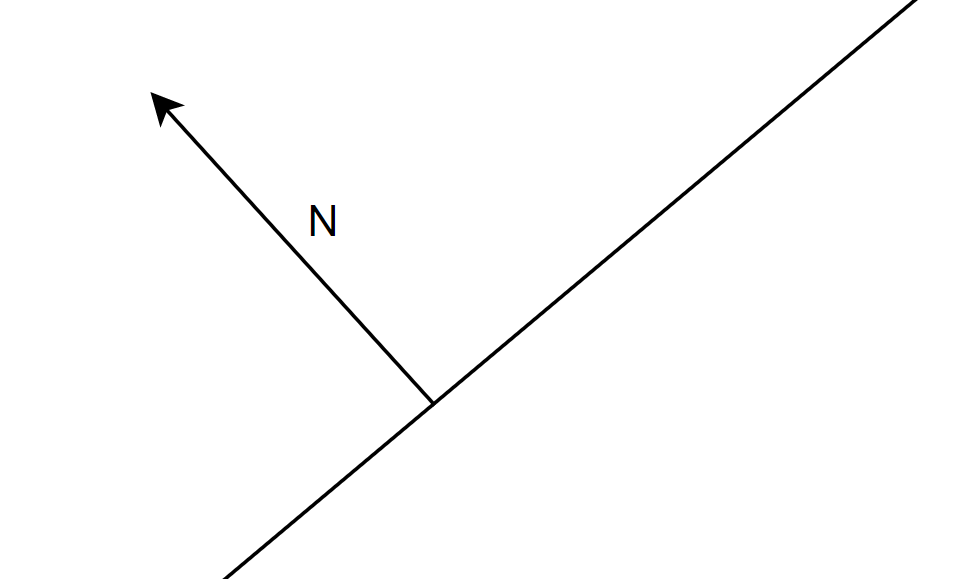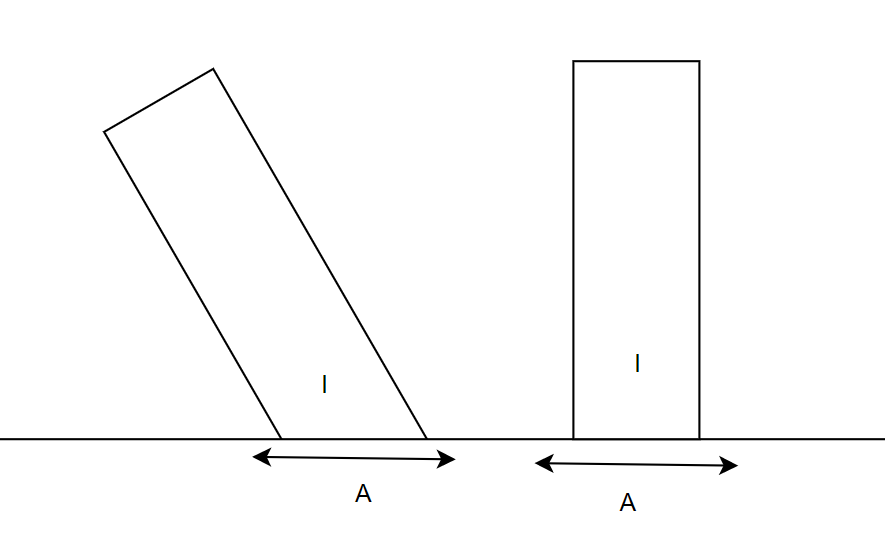# 漫反射

## 简单分析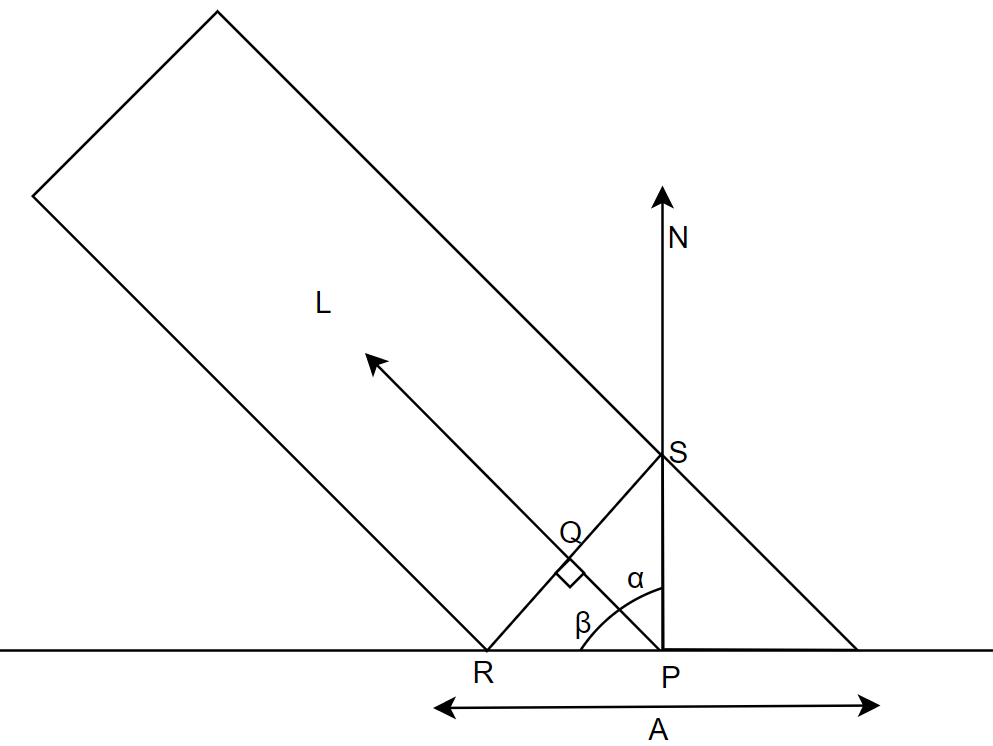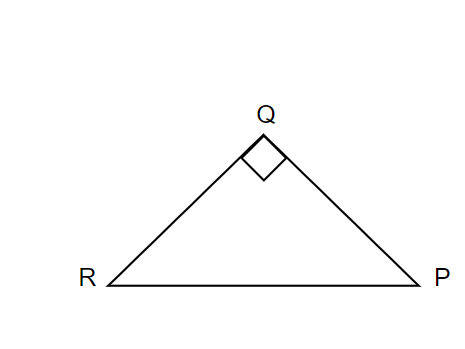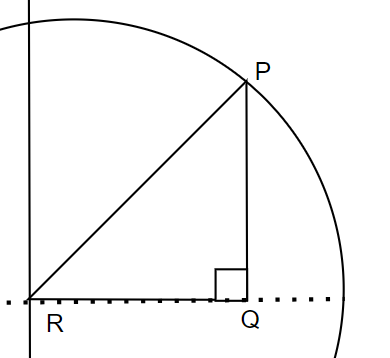cos(a)=RQ/RP=I/2/A/2,分子分母上下乘以一个数值不变，所以上下同时乘以2等于cos（a）=I/A.

I/A=cos(a),这个比值得到了一个函数。

L dot N等于|L| |N|*COS（a）

## 代码

　　　　　class Light
{
public Vector3D Direction
{
get; set;
}
public Vector3D Position
{
get; set;
}
public LightEnum LightType
{
get; set;
}
public double Intenesity
{
get; set;
}
}

       enum LightEnum
{
Ambient,
Point,
Directional
}

定义三个光，强度总量为1

  　　 List<Light> lightlist = new List<Light> {

new Light(){  LightType= LightEnum.Ambient,Intenesity=0.2 },
new Light(){  LightType= LightEnum.Point,Intenesity=0.6,Position=new Vector3D(2,1,0) },
new Light(){  LightType= LightEnum.Directional,Intenesity=0.2,Direction=new Vector3D(1,4,4) }
};

  　　　 /// <summary>
/// 计算光
/// </summary>
/// <param name="p">表面点P</param>
/// <param name="n">法向量</param>
/// <returns>强度</returns>
double ComputeLighting(Vector3D p, Vector3D n)
{
double i = 0;
Vector3D l = new Vector3D();
//遍历所有的光
foreach (var light in lightlist)
{  //不同的写法
if (light.LightType == LightEnum.Ambient)
{
i += light.Intenesity;
}
else
{
if (light.LightType == LightEnum.Point)
{
l = light.Position - p;
}
else
{
l = light.Direction;
}
var dot = Vector3D.DotProduct(n, l);
if (dot > 0)
{
i += light.Intenesity * dot / (n.Length * l.Length);
}
}

}
return i;
}

 　　　　Color tracrray(Vector3D origin, Vector3D dline, double min, double max)
{
double closet = double.PositiveInfinity;
sharp claset_sharp = null;
foreach (var item in sharplist)
{
var gp = IntersectRayShere(origin, dline, item);
if (gp.X >= min && gp.X <= max && gp.X < closet)
{
closet = gp.X;
claset_sharp = item;
}
if (gp.Y >= min && gp.Y <= max && gp.Y < closet)
{
closet = gp.Y;
claset_sharp = item;
}
}
if (claset_sharp == null)
{
return Colors.White;
}
var p = origin + (closet * dline);
var n = p - claset_sharp.center;
n = n / n.Length;
var cl = ComputeLighting(p, n);

return Color.FromRgb((byte)(cl * claset_sharp.color.R), (byte)(cl * claset_sharp.color.G), (byte)(cl * claset_sharp.color.B));
}

# 镜面反射

## 镜面反射分析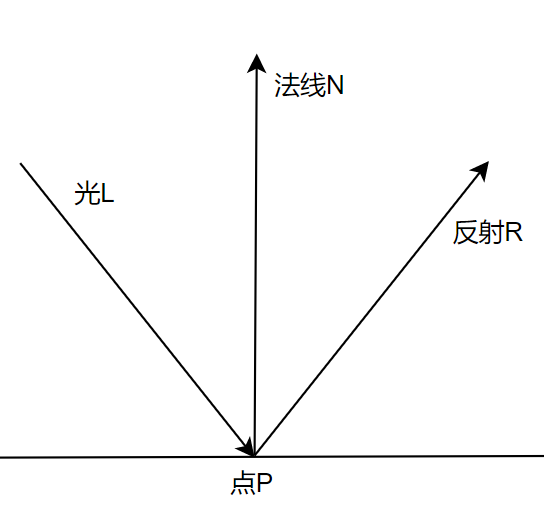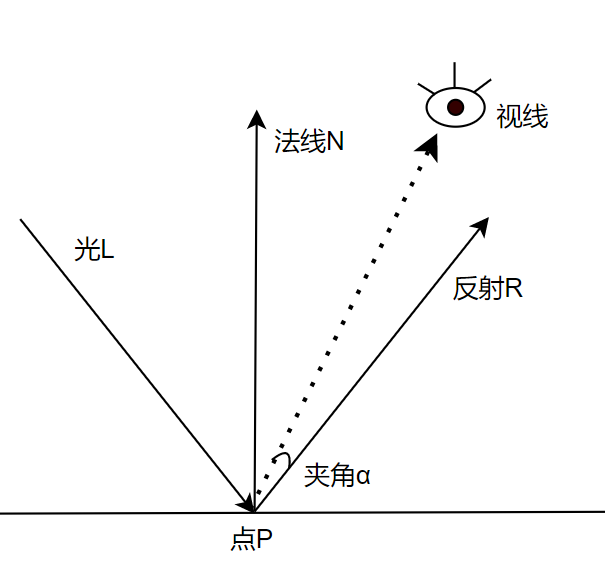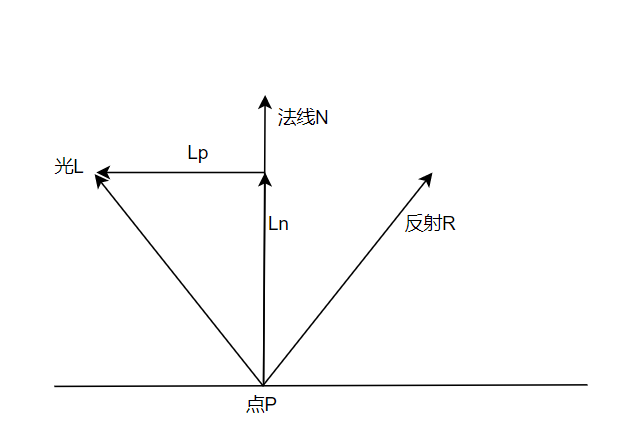求lp必须要求LN，才可以因为ln=l-ln，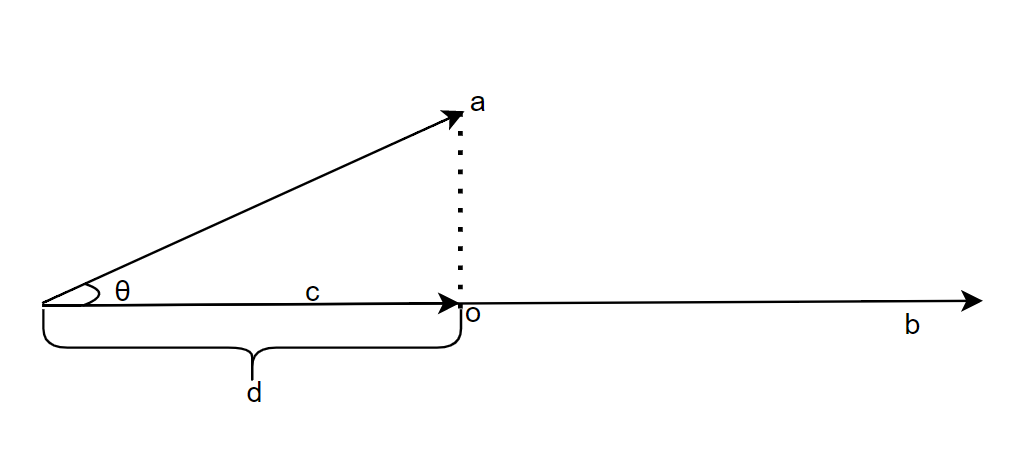$\overrightarrow{c}=d\dfrac{\overrightarrow{b}}{\left| b\right| }$

$d=\left| a\right| \cos \left( \theta \right)$

$\cos \left( \theta \right) =\dfrac{\overrightarrow{a}\cdot \overrightarrow{b}}{\left| a\right| \left| b\right| }$

$\overrightarrow{c}=\left| a\right| \dfrac{\overrightarrow{a}\cdot \overrightarrow{b}}{\left| a\right| \left| b\right| }\dfrac{\overrightarrow{b}}{\left| b\right| }$

$\overrightarrow{c}=\left| a\right| \dfrac{\overrightarrow{a}\cdot \overrightarrow{b}}{\left| a\right| \left| b\right| }\overrightarrow{b}\dfrac{1}{\left| b\right| }$

$\overrightarrow{c}=\dfrac{\overrightarrow{a}\cdot \overrightarrow{b}}{\left| b\right| ^{2}}\overrightarrow{b}$

$R=N(N\cdot L)-(L-N(N \cdot L))$

$R=2N(N \cdot L)-L$

## 光泽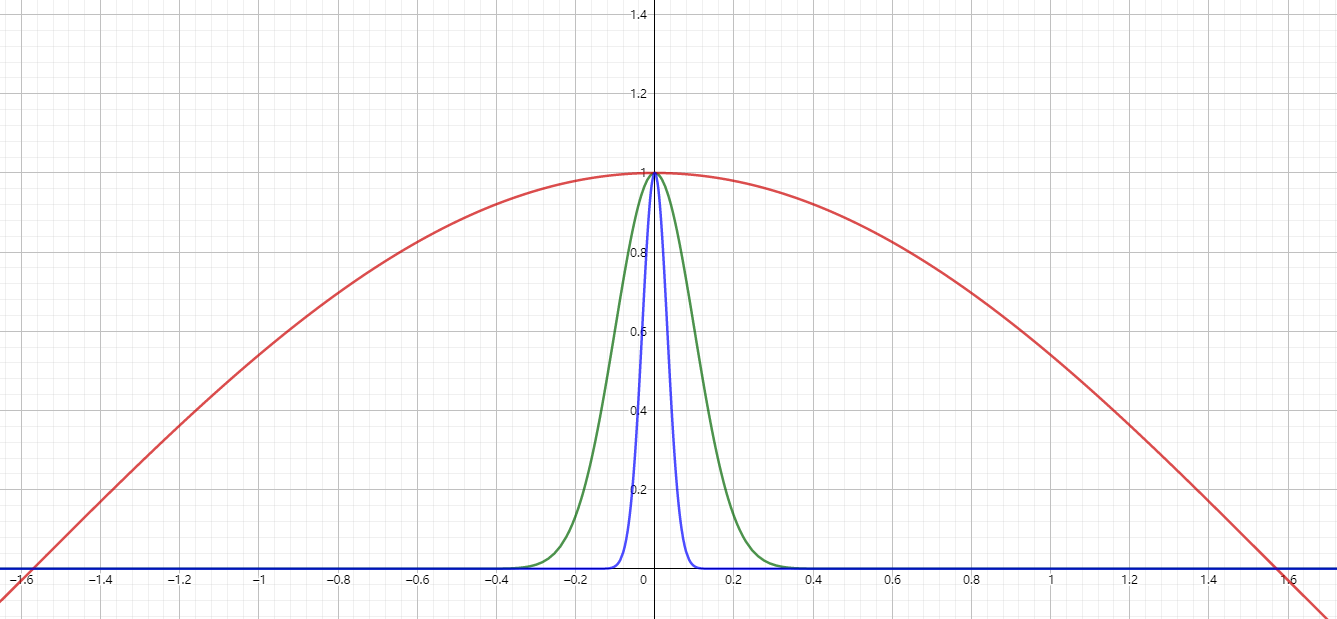cosx的X值越大，突起越明显就以为特闪亮。

镜面反射公式

$\left( \dfrac{\overrightarrow{p}\cdot \overrightarrow{R}}{\left| p\right| \left| R\right| }\right) ^{s}$

## 代码

  　　　　 class sharp
{
public Vector3D center
{
get; set;
}
public double radius { get; set; } = 1;
public Color color
{
get; set;
}
public double specular
{
get; set;
}
}

　　　　 List<sharp> sharplist = new List<sharp>
{
new sharp() { center = new Vector3D(0, -1, 3), color = Colors.Red,specular=500 },
new sharp() { center = new Vector3D(2, 0, 4),  color = Colors.Blue,specular=500 },
new sharp() { center = new Vector3D(-2, 0, 4), color = Colors.Green ,specular=10},
};

     double ComputeLighting(Vector3D p, Vector3D n, Vector3D v, double s)
{
double i = 0;
Vector3D l = new Vector3D();
foreach (var light in lightlist)
{
if (light.LightType == LightEnum.Ambient)
{
i += light.Intenesity;
}
else
{
if (light.LightType == LightEnum.Point)
{
l = light.Position - p;
}
else
{
l = light.Direction;
}
var dot = Vector3D.DotProduct(n, l);
if (dot > 0)
{
i += light.Intenesity * dot / (n.Length * l.Length);
}
if (s != -1)
{
var r = 2 * Vector3D.DotProduct(n, l) * n - l;
var dotv = Vector3D.DotProduct(r, v);
if (dotv > 0)
{
i += light.Intenesity * Math.Pow(dotv / (r.Length * v.Length), s);
}
}
}

}
//可以设置大1为1
//i>1?1:i
return i;
}

  Color tracrray(Vector3D origin, Vector3D dline, double min, double max)
{
double closet = double.PositiveInfinity;
sharp claset_sharp = null;
foreach (var item in sharplist)
{
var gp = IntersectRayShere(origin, dline, item);
if (gp.X >= min && gp.X <= max && gp.X < closet)
{
closet = gp.X;
claset_sharp = item;
}
if (gp.Y >= min && gp.Y <= max && gp.Y < closet)
{
closet = gp.Y;
claset_sharp = item;
}
}
if (claset_sharp == null)
{
return Colors.Transparent;
}
var p = origin + (closet * dline);
var n = p - claset_sharp.center;
n = n / n.Length;
var cl = ComputeLighting(p, n, -dline, claset_sharp.specular);
//保证颜色上下限正确
var M = Color.FromRgb((byte)(Math.Min(255, Math.Max(0, cl * claset_sharp.color.R))),
(byte)(Math.Min(255, Math.Max(0, (cl * claset_sharp.color.G)))),
(byte)(Math.Min(255, Math.Max(0, (cl * claset_sharp.color.B)))));

return M;
}

posted @ 2022-06-27 16:24  ARM830  阅读(37)  评论(0编辑  收藏  举报